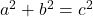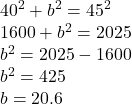## A cable 45 m long runs from the top of a utility pole to a point on the ground 40 m from the base of the pole. How tall is the utility pole,

Question

A cable 45 m long runs from the top of a utility pole to a point on the ground 40 m from the base of the pole. How tall is the utility pole, to the nearest tenth?
20.6 m

85 m

5 m

60.2 m

in progress 0
6 months 2021-07-27T07:54:05+00:00 1 Answers 3 views 0

20.6 m

Step-by-step explanation:

Hi there!

Assuming that the utility pole makes a 90-degree angle with the ground, this scenario creates a right triangle.

The 45 m-long cable is the hypotenuse and the 40 m between the base of the pole and the cable is one of the legs.

We must solve for the height of the utility pole, which is essentially the other leg of the right triangle.

We can use the Pythagorean theorem:where a and b are the legs and c is the hypotenuse

Plug in the known information (leg=40, hyp=45)Therefore, when rounded to the nearest tenth, the height of the utility pole is 20.6 m.

I hope this helps!